WEBINAR
Efficient Green Energy: Exploring Simulation-Driven Design for High-Torque Wind Turbine Machine
Thursday, September 21, 2023
Time
SESSION 1
SESSION 2
CEST (GMT +2)
03:00 PM
08:00 PM
EDT (GMT -4)
09:00 AM
02:00 PM
HOME / Applications / Modeling-Simulation and Analysis of MEMS microsensing membrane

# Modeling-Simulation and Analysis of MEMS microsensing membrane

Used Tools:## Introduction

Electrostatic actuation, commonly used in MEMS, is based on the electrostatic fields and the forces they generate on the structures.
The deformation of electrodes, caused by the electrostatic forces, is the main concern in electromechanical actuators such as RF micro-switches, comb-drives and Pressure sensors.

In fact, an electric field is created between two electrodes by applying a potential difference which generates electrostatic forces that will be main cause of deflection.

This technology has been used in many industries, such as automotive and bio-medical, due to its light weight, compact size, low-power consumption, and durability.

EMS Simulation software from EMWorks ; has been used to study a resultant deflection of a membrane under an applied dc voltage; the Electrostatic analysis type with coupling to structural has been used to achieve such goal.
In the carried out analysis, the electric force has been considered and the gravity acceleration has been ignored.

## The Model Geometry

Figure 1 and figure 2 show the geometry of the analyzed model. It consist of two 40square membranes, one is fixed at the bottom and a movable one at the top, controlled with two serpentine springs.
The thickness of the device is equal to 1, along z axis.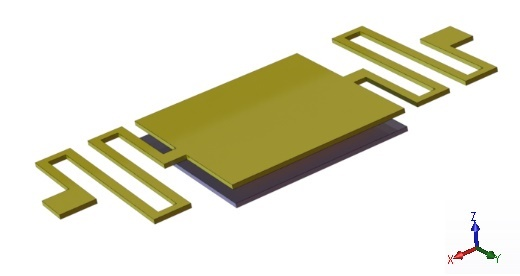Figure 1 - The geometry of the analyzed model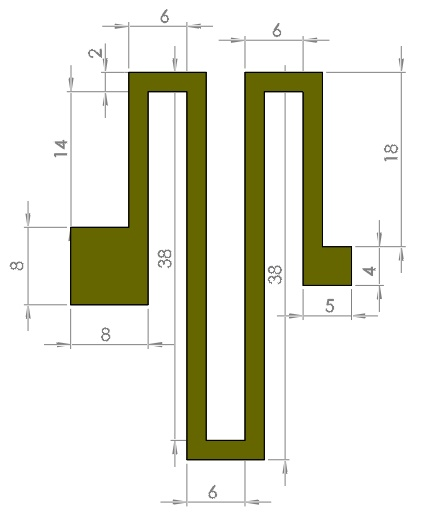Figure 2 - The geometry of the serpentine spring

All the units are given in micrometer.

## Simulation Setup

The Electrostatic module of EMS, coupled to structural analysis, has been used to compute and visualize the electric results and the mechanical deformation of the moving membrane.

To perform an analysis using EMS, the following important steps have been performed:

1. Select an appropriate material for all solid bodies.
2. Define the necessary electromagnetic inputs.
3. Apply the structural boundary conditions.
4. Mesh the entire model and run the solver.

## Materials Properties

Table 1 given below summarizes the required material properties for the simulation.

Table 1 - Properties of materials assigned to the model

 Material Name Relative permittivity Density (kg/) Elastic Modulus (Pa) Poisson’s Ratio Platinum 1 2145 170e+09 0.26 Air 1 0 Not required Not required

## Boundary conditions

### Electrical boundary condition

1. Fixed voltage 1(0V)

The top membrane is grounded, as shown in figure 3. The arrows show the symbols of the boundary condition given to it.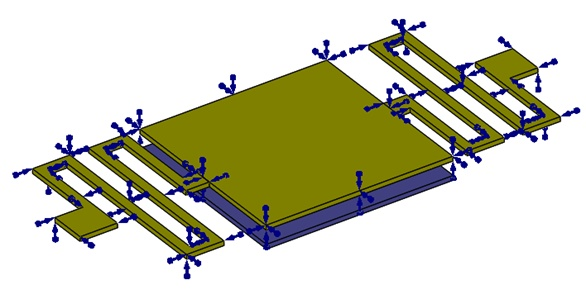Figure 3 - Fixed voltage applied to the top membrane

2. Fixed voltage 2 ( positive voltage)

The fixed electrode is assigned a positive voltage. Figure 4 shows where the voltage is applied.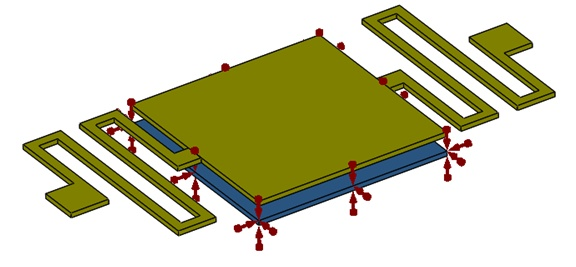Figure 4 - Fixed voltage applied to the bottom membrane

### Structural boundary condition

Fixed boundary conditions are applied to the bottom membrane and to the anchors of the two serpentine springs, as shown in the figure 5: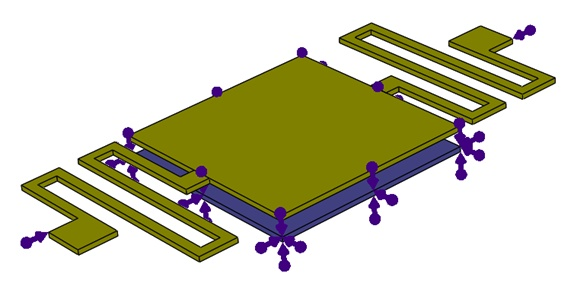Figure 5 - Fixed constraint applied on the bottom membrane

## Meshing

The model geometry doesn’t contain very complicated shapes. A mesh control has been applied to all solid bodies of the mode; this would be sufficient to get accurate electrical and structural results. Figure 6 shows the generated mesh.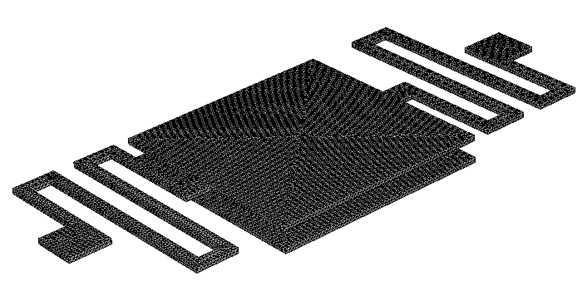Figure 6 - The meshed model

## Simulation Results

Different voltages have been applied to the bottom membrane. The generated electrostatic force is the origin of the top membrane deflection.
The figure 7, shows the resultant displacement as a function of the applied voltage computed by EMS compared with the reference results.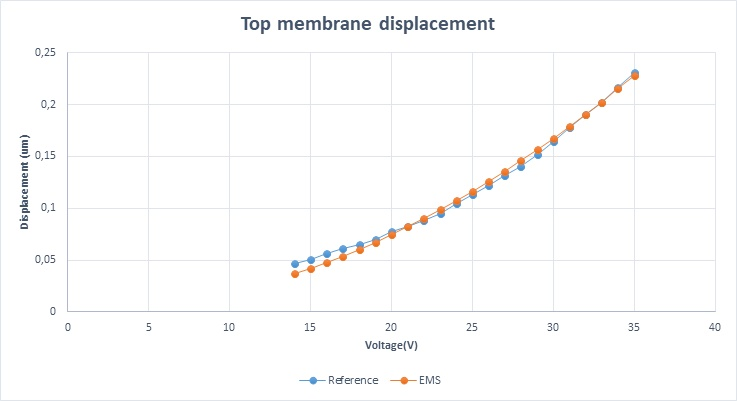Figure 7 - displacement as function of voltage for EMS and reference

### Visualization of the Results for 33v applied Voltage:

EMS offers the possibility to find out the resultant electric rigid body force acting on the structure parts. In our case, we are interested in finding the electric force acting on the top membrane
Table 2 shows the components of the electric force vector acting on the membrane. The force is given in Newtons.

#### Electric force (Results table)

Table 2 - The components of the electric force vector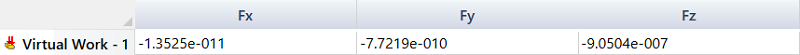The Analytical formulaC: the capacitance
V: the voltage applied to bottom membrane

#### Correlation between EMS and the reference result:

Table 3 - Comparison between EMS and the reference results

 EMS Result Reference Result Resultant Displacement under 33V (in) 0.202591 0.201708

#### Resultant Displacement plot: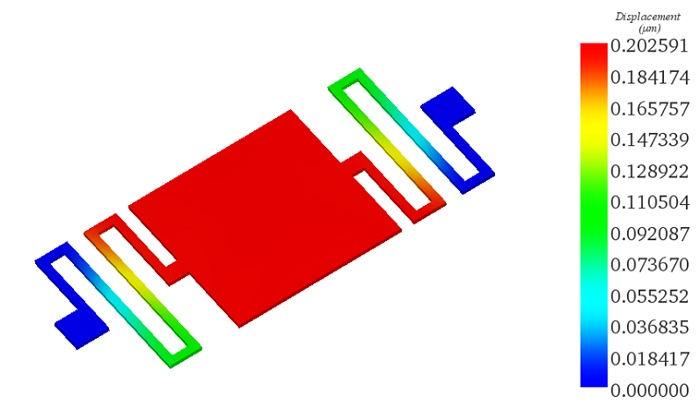Figure 8 - Resultant displacement distribution on the top membrane

Figure 8 shows that the maximum displacement occurs at the square membrane

## Conclusion

The structural behavior of the microsensing membrane with two serpentine springs has been investigated using EMS simulation software. The computed displacement found in a good agreement with the reference results .

This numerical analysis helped to get an idea of how the MEMS device responds to different DC voltage inputs; in the studied case, the displacement of the bottom membrane kept increasing with the increase of the applied voltage.

#### References

: https://www.emworks.com/
: P. A. Manoharan and D. Nedumaran, 2010, “Modeling-Simulation and Analysis of MEMS Capacitive Millibar Pressure Sensor,” J. Nanotechnol. Eng. Med 1(4)